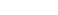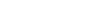Get inspired by the success stories of our students in IIT JAM MS, ISI  MStat, CMI MSc DS.  Learn More

# ISI MStat 2019 PSA Problem 11 | Multiplication PrincipleThis is a beautiful problem from ISI MSTAT 2019 PSA problem 11 based on multiplication principles. We provide sequential hints so that you can try.

## Multiplication Principle - ISI MStat 2019 PSA - 11

How many positive divisors ofare perfect squares?

• 60
• 18
• 120
• 4

### Key Concepts

Basic counting principles

Divisors of a number

ISI MStat 2019 PSA Problem 11

A First Course in Probability by Sheldon Ross

## Try with Hints

See in order to get the positive divisors ofthat are perfect squares , we need to take only the even powers of the primes {2,5,11}.

Now the maximum powers of 2 is 5 . So there are 3 choices for even powers {0,2,4} .

The maximum powers of 5 is 3 . So there are 2 choices for even powers {0,2}.

Now the maximum powers of 2 is 5 . So there are 3 choices for even powers {0,2,4} .

The maximum powers of 11 is 4 . So there are 3 choices for even powers {0,2,4}.

Hence by multiplication principle we have in totalsuch positive divisors.

## Subscribe to Cheenta at Youtube

This is a beautiful problem from ISI MSTAT 2019 PSA problem 11 based on multiplication principles. We provide sequential hints so that you can try.

## Multiplication Principle - ISI MStat 2019 PSA - 11

How many positive divisors ofare perfect squares?

• 60
• 18
• 120
• 4

### Key Concepts

Basic counting principles

Divisors of a number

ISI MStat 2019 PSA Problem 11

A First Course in Probability by Sheldon Ross

## Try with Hints

See in order to get the positive divisors ofthat are perfect squares , we need to take only the even powers of the primes {2,5,11}.

Now the maximum powers of 2 is 5 . So there are 3 choices for even powers {0,2,4} .

The maximum powers of 5 is 3 . So there are 2 choices for even powers {0,2}.

Now the maximum powers of 2 is 5 . So there are 3 choices for even powers {0,2,4} .

The maximum powers of 11 is 4 . So there are 3 choices for even powers {0,2,4}.

Hence by multiplication principle we have in totalsuch positive divisors.

## Subscribe to Cheenta at Youtube

This site uses Akismet to reduce spam. Learn how your comment data is processed.

### Knowledge Partner